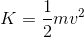AP Physics C Electricity : Mechanics Exam

Example Questions

Example Question #1 : Interpreting Linear Motion Diagrams

A guillotine blade weighing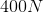is accelerated upward into position at a rate of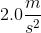.

What is the the approximate mass of the guillotine blade?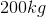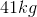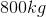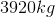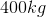Explanation:

The force of gravity on the blade is, which is the same as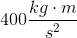This unit relationship comes from Newton's second law.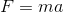is the mathematical expression of Newton's second law. The units for force must be a product of the units for mass and the units for acceleration.

Solve the expression by plugging in known values.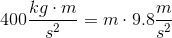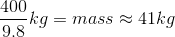Example Question #1 : Interpreting Linear Motion Diagrams

A guillotine blade weighingis accelerated upward into position at a rate of.

What is the tension on the rope pulling the blade, while it is accelerating into position?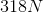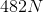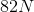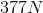Explanation:

The tension in the rope is the sum of the forces acting on it. If one considers that the net force on an object must equal the mass of the object times the acceleration of the object, the net force on the object must be the force due to tension from the rope minus the force due to gravity.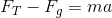Rearrange the equation.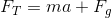Plug in known values.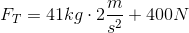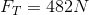Example Question #21 : Mechanics Exam

Two objects moving in one dimension created the following velocity vs. time graph: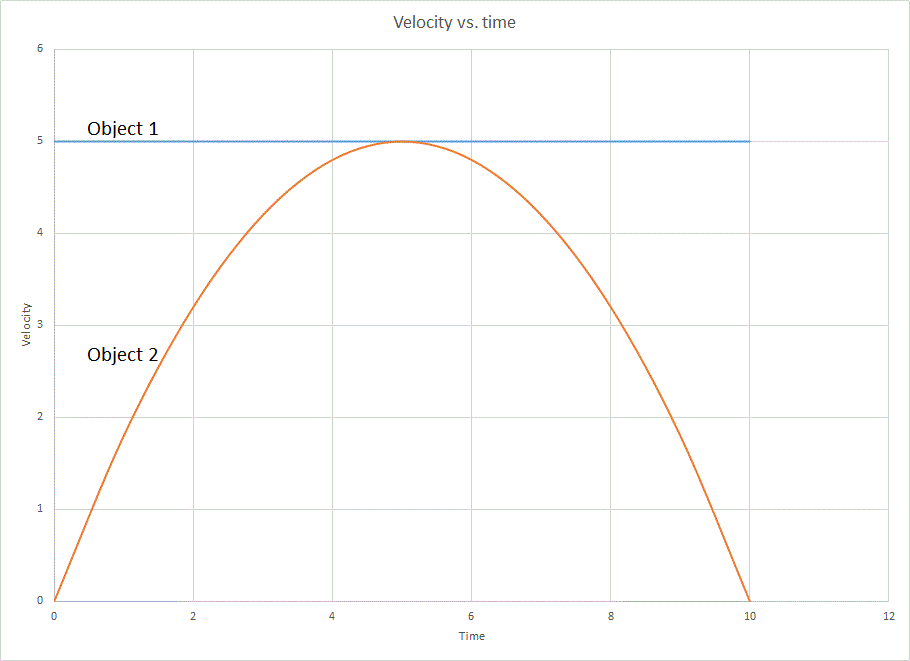From the graph above, what is true about the two objects at time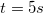?

They have travelled the same distance from their starting positions

They are moving at the same speed

They are at the same position

They are both at rest (not moving)

One object is passing the other

They are moving at the same speed

Explanation:

Since this is a graph of velocity and not position, the curves intersect where the velocities match. Since we do not know the starting position, we do not know where the objects are relative to one another.

Example Question #2 : Interpreting Linear Motion Diagrams

Two objects moving in one dimension created the following velocity vs. time graph:From the graph above, which object has traveled a greater distance from its starting position when?

Object 1

They have travelled an equal distance

Object 2

It cannot be determined from the graph

None of these

Object 1

Explanation:

Since this is a graph of velocity vs. time, its integral is distance travelled. We can estimate the integral by looking at the area under the curves. Since Bbject 1 has a greater area under its velocity curve, it has covered a greater distance. Its velocity is greater that Object 2's for the entire time, so it makes sense that it will travel farther.

Example Question #2 : Interpreting Linear Motion Diagrams

An object is moving in two dimensions. Its vertical motion relative to the horizontal motion is described by the equation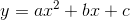. Its motion in the horizontal direction is described by the equation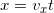. What is the object's velocity is thedirection in terms of its horizontal position?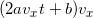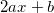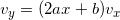Explanation:

The y velocity is the time derivative of theposition, and not thederivative. In order to find it, use the chain rule: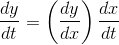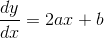Of course,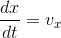Example Question #6 : Interpreting Linear Motion Diagrams

Atwood's machine consists of two blocks connected by a string connected over a
pulley as shown. What is the acceleration of the blocks if their masses are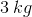and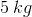.

Assume the pulley has negligible mass and friction.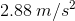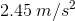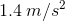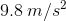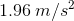Explanation: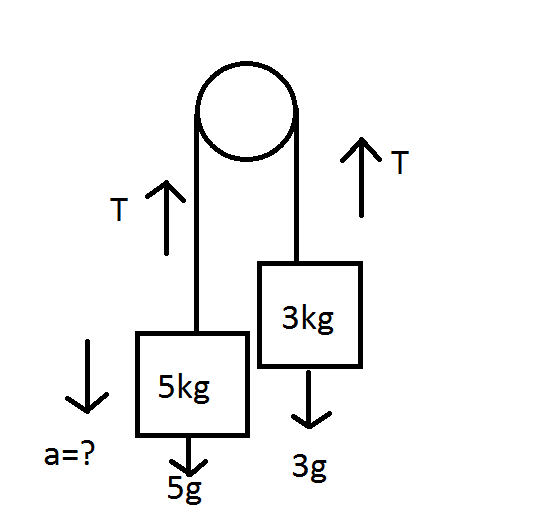From the force diagram above, we can see that tension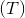is pulling up on both sides of the string and gravity is pulling down on both blocks. With this information we can write 2 force equations: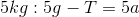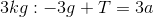If we add the two equations together, we get: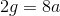where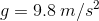Solving for, we get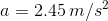Example Question #1 : Harmonic Motion

A 5.0 kg mass oscillates once. The total distance it travels is 1.5 m and it takes 4.0 s to travel that distance. What is its frequency of oscillation?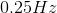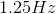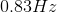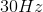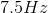Explanation:

Frequency is only based on the period of the oscillation; all the other given information is useless for this problem.  Using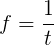, we can calculate that the frequency is 0.25 Hz.

Example Question #21 : Ap Physics C

A mass oscillates on a spring with period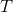. If the mass is doubled, what is the new period of oscillation?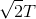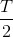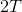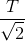Explanation:

The formula for the period of oscillation is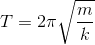.

When we double the mass, we get: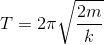Because the new factor of 2 is under the square root sign, and also in the numerator, the new period will be increased by.

Example Question #1 : Understanding Principles Of Harmonic Motion

A mass is attached to the ceiling by a spring and hangs vertically downward. The mass is stretched downward so its amplitude isunits from its equilibrium position. What is the distance the mass travels during one full oscillation?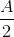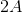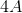Explanation:

When the mass is at point, it hasn't traveled at all. When it reaches the spring's equilibrium point, it has traveled a distance of. The mass then continues to a point that's equal to the initial distance traveled, but on the opposite side of the equilibrium point, so the total distance traveled so far is. The mass must then travel back to the starting point to complete the oscillation, so the total distance traveled is.

Example Question #21 : Mechanics Exam

A mass is attached to a spring, which is fixed to a wall. The mass is pulled away from the spring's equilibrium point and is then released. At what point does the mass experience its maximum kinetic energy?

Halfway through one full oscillation

At the spring's equilibrium point

The mass does not have kinetic energy

Equal in all places

Where the mass started